# Learn Subtraction Using a Number Line

1. Math Lessons >
2. Number Line Subtraction

### Overview of Subtraction on a Number Line:

Are you looking for effective tools to help your kids understand subtraction? With number lines around, you don’t need to shop elsewhere. In this lesson, let’s learn to subtract whole numbers using a number line.

### How Do We Subtract Numbers on a Number Line?

Let’s subtract 3 from 5. We have our number line and Skittles waiting at 5.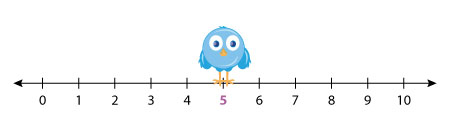Enliven her with a click!

Our little birdie starts from 5, hops backward three times, and lands on the number 2.

So, 5 – 3 = 2.

Let’s move on to our next problem: 9 – 4. This time we have Mr. Snowball on the number line.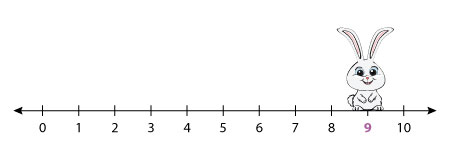Activate him with a click!

He starts from 9, hops 4 steps backward, and lands on 5, which is our answer.

So, we can say 9 – 4 = 5.

### How Do We Find a Missing Minuend or Subtrahend Using a Number Line?

Let’s recall that in a subtraction sentence, the first number is the minuend, the second number that is taken away from the first is the subtrahend, and the number obtained as a result is the difference.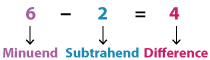It’s pretty simple to find a missing minuend or subtrahend of a subtraction equation modelled on a number line. Observing the above number lines, we can say:

Hop backward as many times as the subtrahend.

Land at the difference.

So, you can easily figure out the missing minuend by locating the starting point and the missing subtrahend by counting the hops. Let’s complete the subtraction sentence 11 – _____ = 3 using this model.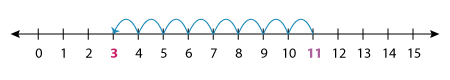How many hops are drawn? The answer to this question is the missing subtrahend.

Done with counting? Yes, you’re right! It’s 8.

Thus, the subtraction equation is 11 – 8 = 3.

1) What is 15 – 8?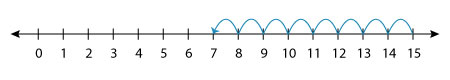Thus, we have 15 – 8 = 7.

2) Which subtraction sentence does the number line represent?The number line represents 8 – 8 = 0.

3) Which number is subtracted from 13 so we get 7?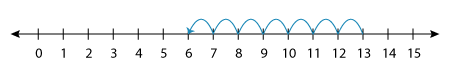We get 7 when we subtract 6 from 13.Whizz through subtracting numbers using our free printable Subtraction with Number Lines worksheets!

Progress
Score

Restart Quiz